# 特殊方法 | Python从入门到精通：高阶篇之三十六

## 特殊方法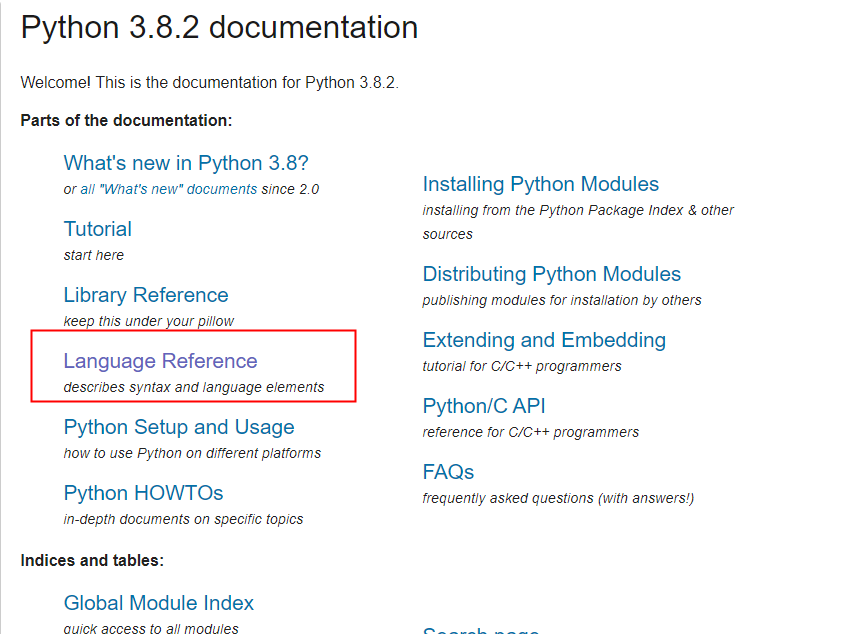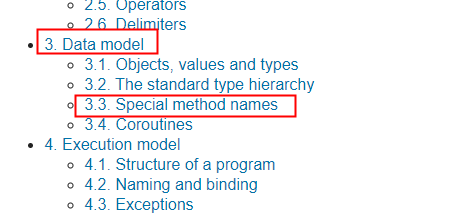class Person(object):
"""人类"""
def __init__(self, name , age):
self.name = name
self.age = age

# 创建两个Person类的实例
p1 = Person('孙悟空',18)
p2 = Person('猪八戒',28)

# 打印p1
print(p1)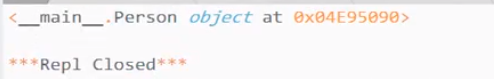t = 1,2,3
print(t)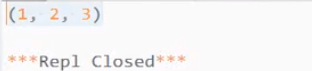__str__()：

    def __str__(self):
return 'Hello Person'

print(p1)
print(p2)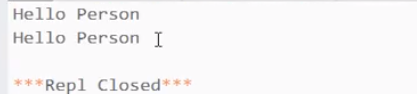def __str__(self):
return 'Person [name=%s , age=%d]'%(self.name,self.age)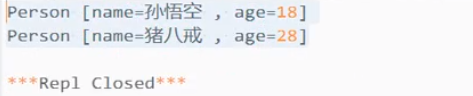__str__（）这个特殊方法会在尝试将对象转换为字符串的时候调用，它的作用可以用来指定对象转换为字符串的结果 （print函数）。

__repr__()：

    def __repr__(self):
return 'Hello'

print(repr(p1))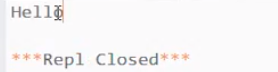__repr__()这个特殊方法会在对当前对象使用repr()函数时调用，它的作用是指定对象在 ‘交互模式’中直接输出的效果。

print(p1 > p2)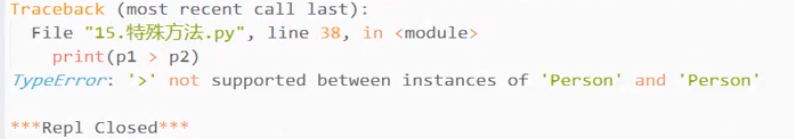object.__lt__(self, other)    #  小于 <
object.__le__(self, other)   # 小于等于 <=
object.__eq__(self, other)     # 等于 ==
object.__ne__(self, other)    # 不等于 !=
object.__gt__(self, other)     # 大于 >
object.__ge__(self, other)    # 大于等于 >= 

    def __gt__(self , other):
return True

print(p1 > p2)
print(p2 > p1)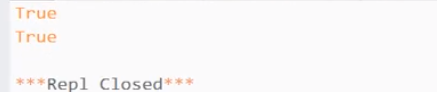return self.age > other.age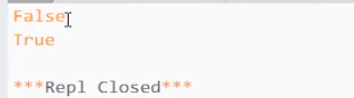__gt__会在对象做大于比较的时候调用，该方法的返回值将会作为比较的结果。他需要两个参数，一个self表示当前对象，other表示和当前对象比较的对象。简单来说：self > other

__len__()：获取对象的长度

object.__bool__(self)

    def __bool__(self):
return self.age > 17

print(bool(p1))p1 = Person('孙悟空',8)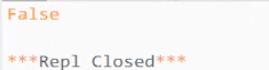if p1 :
print(p1.name,'已经成年了')
else :
print(p1.name,'还未成年了')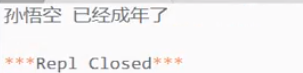object.__add__(self, other)
object.__sub__(self, other)
object.__mul__(self, other)
object.__matmul__(self, other)
object.__truediv__(self, other)
object.__floordiv__(self, other)
object.__mod__(self, other)
object.__divmod__(self, other)
object.__pow__(self, other[, modulo])
object.__lshift__(self, other)
object.__rshift__(self, other)
object.__and__(self, other)
object.__xor__(self, other)
object.__or__(self, other)      

### 配套视频课程，点击这里查看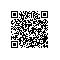Python学习资料大全，包含Python编程学习、实战案例分享、开发者必知词条等内容。